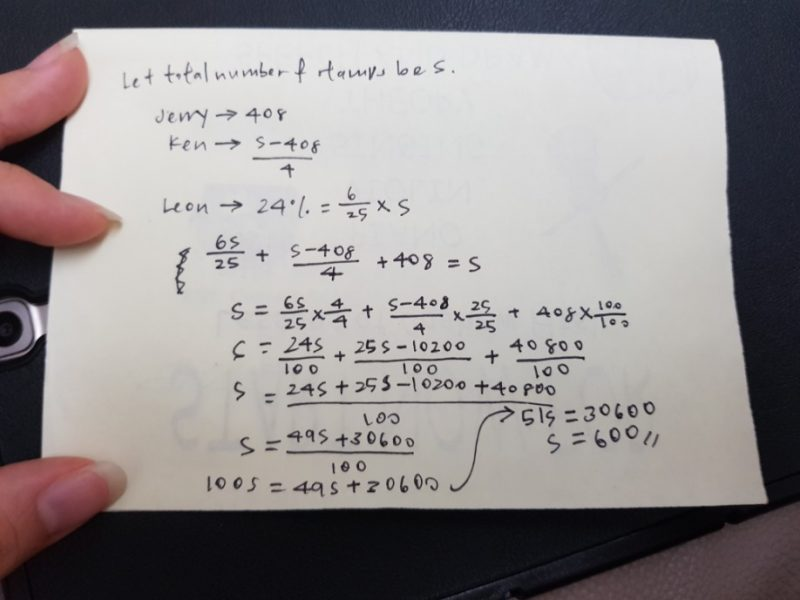# Question0 Replies 0 Likes

L took 3/4 of remainder =>24% of total

K took 1/4 of remainder =>24%/3 of total = 8% of total

Total L and K tool => 24% + 8% = 32% of total

No of stamp J took will be

100% – 32% = 408

68% = 408

100% = 408/68 x 100 = 600

and: three boys have 600 stamps altogether.

0 Replies 0 Likes